Home > CC2MN > Chapter cc27 > Lesson cc27.1.8 > Problem7-93

7-93.
1. For each equation below, solve for x. Show the process you use to check your answer. Homework Help ✎

1. x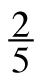= 1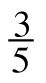2. 5x = 0

3. x − 14.6 = 2.96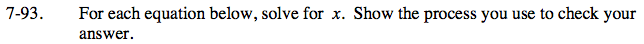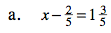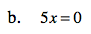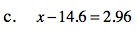Isolate the variable and constants.

$x-\frac{2}{5}=1\frac{3}{5}$

$\;\;\;+\frac{2}{5}\;\;\;+\frac{2}{5}$

$\textit{x}=\frac{8}{5}+\frac{2}{5}$

x = 2